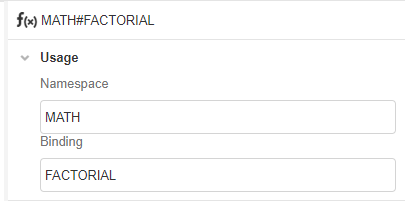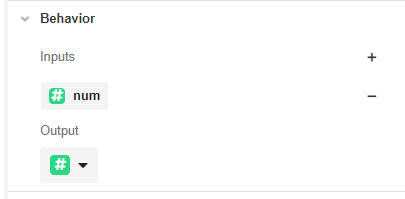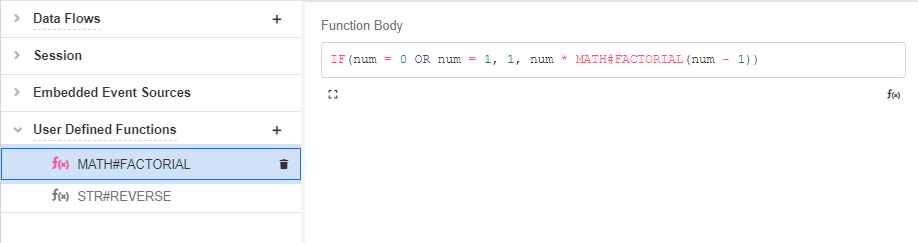# Creating Custom Functions

Creating Custom Functions: "User Defined Functions"

This guide walks through the process of creating two new User Defined Functions. The first example takes a Number as input, cubes it, and returns the absolute value of the cubed result. The second example takes a Number as input and uses recursion to compute its factorial.

# A UDF without recursion

UDFs are created from Connections Builder by clicking on the '+' icon next to User Defined Functions in the Tree:For this example, under the Usage section in the Inspector, define the Namespace value as "ABS_CUBED" and leave the Binding value as "USER_FUNCTION".Then rename the function in the Tree so that it corresponds to how the function will be called upon within the application. User Defined Functions are designated as Namespace#Binding, so this function will be renamed "ABS_CUBED#USER_FUNCTION":Under the Behavior section in the Inspector, define the expected input. `ABS_CUBEB#USER_FUNCTION` will require only a Number as a single input. Click in the '+' icon under Inputs and select Number from the dropdown menu. Label this Number variable "n":Since `ABS_CUBED#USER_FUNCTION` will output a Number, select "Number" from the dropdown menu under Output:In the Function Body that appears in the Stage, use Airscript to define the output of `ABS_CUBED#USER_FUNCTION` in terms of the given input. The following code utilizes the Airscript function ABS as well as the multiplication operator in order to return the absolute value of the cube of the number n:

``````ABS(
n * n * n
)
``````

Inserted in the Function Body, this will appear as follows:We have now created a function, `ABS_CUBED#USER_FUNCTION`, that takes a Number as input, cubes it, and returns the absolute value of the cubed result. Upon saving, this custom function will be accessible in other parts of the Studio, to be called on like any other Airscript function.

# A UDF using recursion

UDFs allow recursion, meaning that a UDF can call on itself recursively. To present a simple case, we’ll create a UDF that mimics the FACT function.

We’ll start by creating a new UDF in Connections Builder and changing the Namespace and Binding under Usage as follows:The function will be automatically renamed to "MATH#FACTORIAL".

This UDF will also receive and retrieve numbers. Therefore, in the Behavior section, add a Variable of type Number and call it num. Then select type Number as the Output.In Function Body, we’ll use the following Airscript function to get the factorial of the Variable "num".

``````IF(num = 0 OR num = 1, 1, num * MATH#FACTORIAL(num - 1))
``````Upon saving, this custom function will be accessible in other parts of the Studio, to be called on like any other Airscript function.